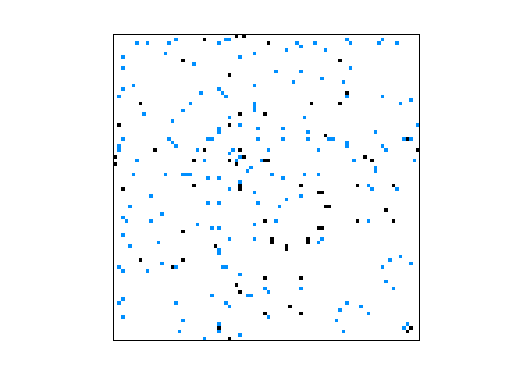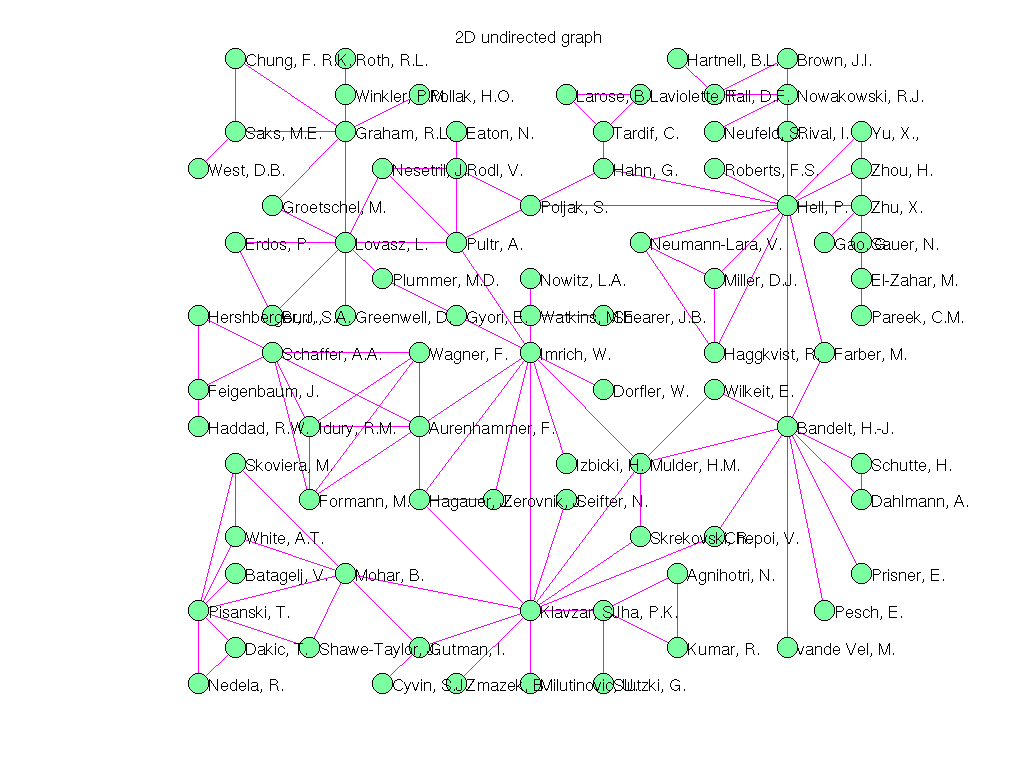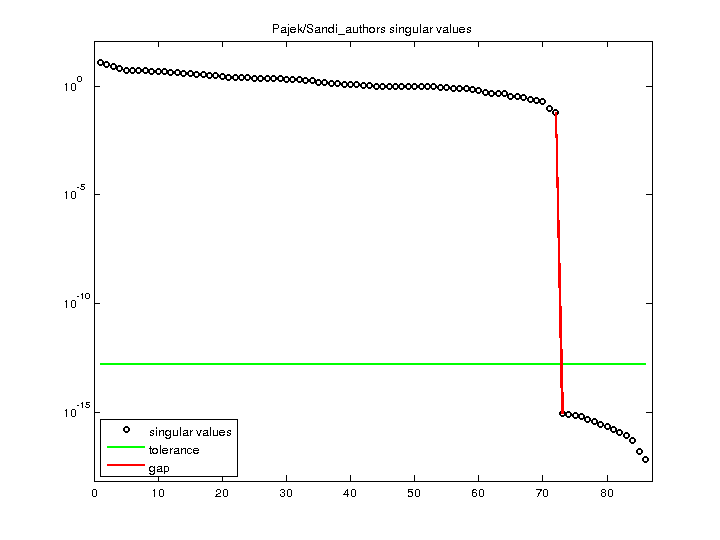Matrix: Pajek/Sandi_authors

Description: Pajek network: Klavzar bibliography(undirected graph drawing)• Matrix group: Pajek
• download as a MATLAB mat-file, file size: 3 KB. Use UFget(1519) or UFget('Pajek/Sandi_authors') in MATLAB.

 Matrix properties number of rows 86 number of columns 86 nonzeros 248 # strongly connected comp. 1 explicit zero entries 0 nonzero pattern symmetry symmetric numeric value symmetry symmetric type integer structure symmetric Cholesky candidate? no positive definite? no

 author I. Klavzar editor V. Batagelj date 1999 kind undirected weighted graph 2D/3D problem? no

 Additional fields size and type nodename full 86-by-16 coord full 86-by-2

Notes:

```------------------------------------------------------------------------------
Pajek network converted to sparse adjacency matrix for inclusion in UF sparse
matrix collection, Tim Davis.  For Pajek datasets, See V. Batagelj & A. Mrvar,
------------------------------------------------------------------------------
The original problem had 3D xyz coordinates, but all values of z were equal
to 0.5, and have been removed.  This graph has 2D coordinates.
```

 SVD-based statistics: norm(A) 12.1011 min(svd(A)) 6.77646e-18 cond(A) 1.78576e+18 rank(A) 72 null space dimension 14 full numerical rank? no singular value gap 7.51165e+13

 singular values (MAT file): click here SVD method used: s = svd (full (A)) ; status: ok